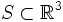# Regular surface

A regular surface in$\R^3$ is a subset$S \subset \R^3$ satisfying the following equivalent conditions:
• It is a two-dimensional differential manifold, embedded inside$\R^3$
• There is an open subset$U \subset \R^3$ containing it, and a smooth map from$U$ to$\R$ under which$S$ is the inverse image of a regular value
It is a theorem that any 2-dimensional compact connected orientable differential manifold can be realized as a regular surface inside$\R^3$, and conversely, any compact regular surface in$\R^3$ is orientable.